Over a million developers have joined DZone.{{announcement.body}}
{{announcement.title}}

DZone's Guide to

# From R-CNN to Faster R-CNN: The Evolution of Object Detection Technology

Let's look at a tutorial that explains how to use object detection to solve the problem of determining where and what an object is.

· AI Zone ·
Free Resource

Comment (0)

Save
{{ articles.views | formatCount}} Views

Bias comes in a variety of forms, all of them potentially damaging to the efficacy of your ML algorithm. Read how Alegion's Chief Data Scientist discusses the source of most headlines about AI failures here.

As per my understanding, the goal of object detection is to find the location of an object in a given picture accurately and mark the object with the appropriate category. To be precise, the problem that object detection seeks to solve involves determining where the object is, and what it is.

However, solving this problem is not easy. Unlike the human eye, a computer processes images in two dimensions. Furthermore, the size of the object, its orientation in the space, its attitude, and its location in the image can all vary greatly.

From the task of image recognition, let's consider an image task. In this task, we need to identify the object in the figure and use a box to indicate its location.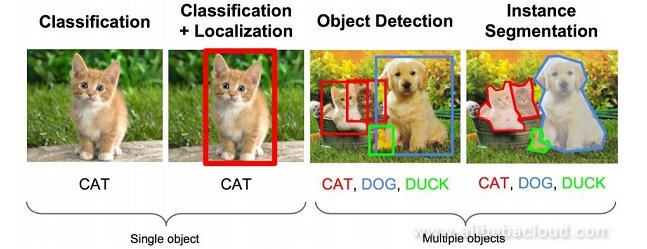Technically, the above task involves image recognition and positioning.

Image recognition (classification)

1. Input: image
2. Output: the type of object
3. Method of evaluation: accuracy

Positioning

1. Input: image
2. Output: the location of the box in the image (x, y, w, h)
3. Method of evaluation: evaluation function intersection-over-union (IOU)

The infamous Convolutional Neural Network (CNN) technology has helped us to complete the task of image recognition. However, we still need to add some additional functions to complete the task of positioning; this is where deep-learning comes into play.

In this article, we will discuss the evolution of object detection technology from the perspective of object positioning. Here's how we can briefly denote the evolution of object detection technology: R-CNN -> SppNET -> Fast R-CNN -> Faster R-CNN

Before we begin, let us take a closer look at Region-based Convolutional Neural Networks (R-CNN).

## Approaching Positioning as a Matter of Regression

If we look at this as a matter of regression, then we need to predict the values of the four parameters (x,y,w,h) to get the position of the box.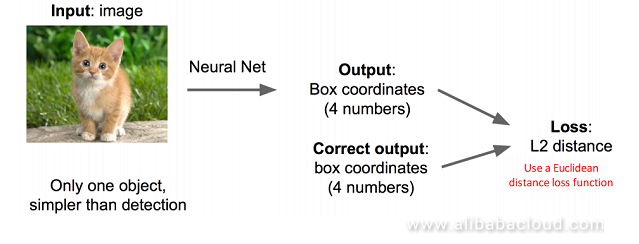Step 1

1. Solve the simplest problem first; use a neural network to identify images
2. Fine-tune on AlexNet VGG GoogleLenet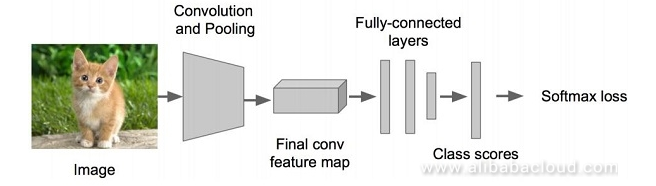Step 2

1. At the tail of the above neural network (that is, before CNN remains unchanged, we make improvements to the end of the CNN; add two headers: "Category Header" and "Regressor Header").
2. Turn it into a classification + regression model.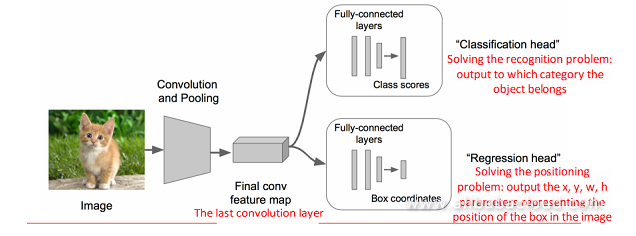Step 3

1. Apply regression using Euclidean distance loss
2. Use SGD training

Step 4

1. Combine the two headers in the prediction phase
2. Complete various functions

Now we need to perform two fine-tuning operations. We can perform the first round on ALexNet, while in the second operation, we will convert the head to a regression head. The front remains unchanged, and we will just have to fine-tune it.

Where to add the regression?
There are two available methods:

1. Add it after the last convolutional layer (e.g. VGG)
2. Add it after the last fully connected layer (e.g. R-CNN)

Performing the regression is quite difficult, so we need to find a way to turn this into a matter of classification. The time to merge training parameters is much longer for regression, so the network above uses a classification network to calculate the link weights of different parts of the network.

## Obtaining the Image Window

1. Use the classification + regression concept from before
2. We first extract boxes of different sizes
3. We put the box in different locations, and then obtain the points for the box in each location
4. Take the box with the highest score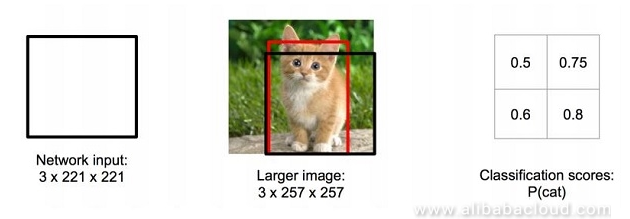Black box in the upper left: score 0.5
Black box in the upper right: score 0.75
Black box in the lower left: score 0.6
Black box in the lower right: score 0.8

According to the scores, we select the lower right box as a prediction of the location of the target.

Note: Sometimes, we select the two boxes with the highest scores, then take the intersection of the two boxes as the final position prediction.

Uncertainty: How big is the box?
After taking different boxes, we first sweep from the upper left corner to the lower right corner. This method is incredibly crude.

Let's sum up the thought process:

For the first image, we use boxes of various sizes (traversing the entire image) to traverse the image and input it to the CNN. Then, the CNN will output a classification of the box and then x, y, w, h of the image in the box (regression).This method is too time-consuming to be considered an optimization. The original network is as follows: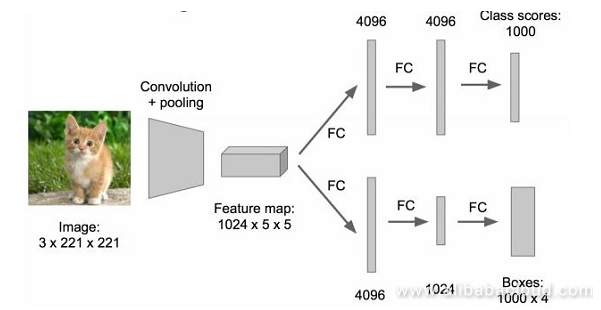To optimize it, change the fully connected layer to a convolution layer to increase speed.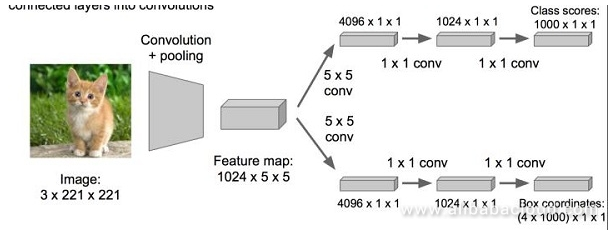## Object Detection

What do we do when there are many objects in the image? In an instant, the difficulties of the task are exponentially magnified. Now the task has become: multi-object recognition + positioning the multiple objects

So can we consider this as a classification task?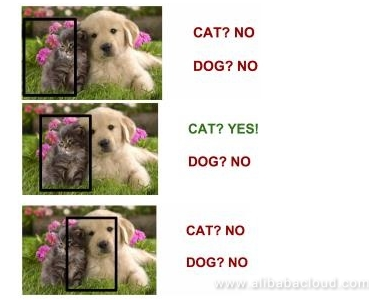What is wrong with seeing it as a classification problem?

1. You need to find a lot of locations and provide several boxes of different sizes
2. You also need to classify the images in the boxes
3. Of course, if your GPU is mighty, well, good luck!

If it's a matter of classification, then how can we go about optimization? I don't want to test so many different locations! Of course, there is a solution to this problem.

First, we find boxes that may contain objects (that is, 1000 or more candidate boxes). These boxes may overlap so that we can avoid all boxes with heavy enumeration.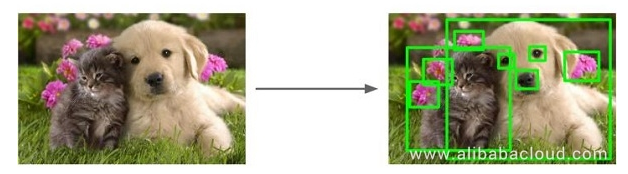There are many methods for selecting candidate frames, such as EdgeBoxes and Selective Search. The following is a comparison of the performance of various methods for selecting candidate frames.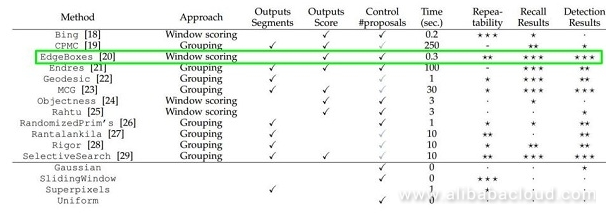Of course, there is not much consensus over how to select these candidate boxes in the "selective search" algorithm used in the extraction of candidate boxes.

## The Arrival of R-CNN

The above ideas fueled the development of R-CNN. Let's look at an implementation of R-CNN with the same image.

Step 1
Train (or download) a classification model (like AlexNet).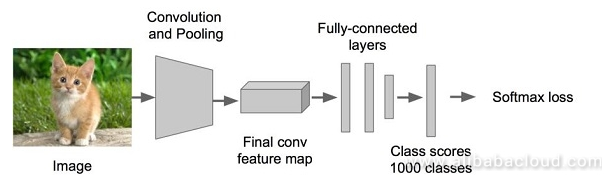Step 2
Fine-tune the model

1. Change the number of categories from 1000 to 20.
2. Remove the last full-connection layer.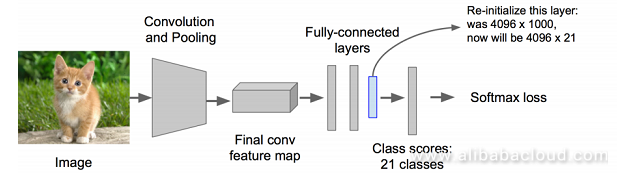Step 3
Feature extraction:

1. Extract all candidate boxes from the image (selective search).
2. For each region: Correct the size of the region to fit the CNN input, do a forward operation, and output the fifth pooled layer (that is, the candidate box extracted features) to the hard disk.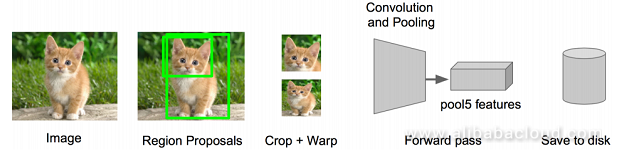Step 4

1. Train an SVM classifier (2 categories) to determine the category of objects in this candidate box.
2. Determine whether the SVM belongs to each of the categories. If so the result is positive, and negative if not. For example, the following figure is the SVM for a dog classification.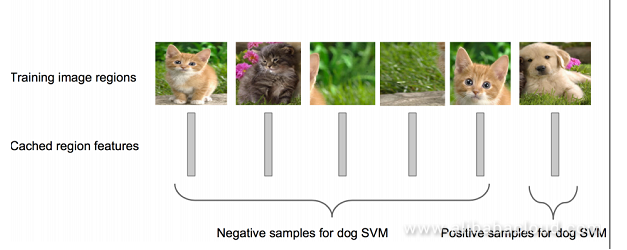Step 5
Use regression to fine-tune the position of the candidate boxes. For each class, train a linear regression model to determine if the box is optimized.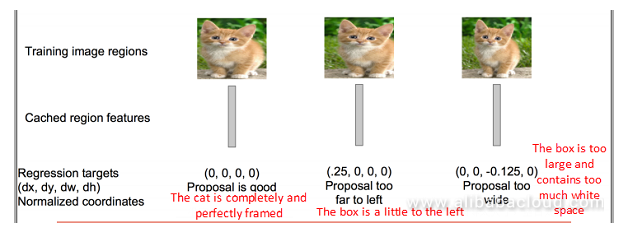## SPP Net

The idea of Spatial Pyramid Pooling (SPP) has made a significant contribution to the evolution of R-CNN. Here we will give a brief introduction of SPP Net.
SPP has two characteristics:

1. Integrate the spatial pyramid method to provide scale input to the CNNs
A full connection layer or classifier follow General CNN. They all need a fixed input size, so they have to crop or warp the input data. This preprocessing can cause data loss or geometric distortion. The first contribution of SPP Net is the addition of the idea of a pyramid to CNN to achieve multi-scale data input.

As shown in the image below, an SPP layer is added between the convolutional layer and the fully connected layer. Now, the input to the network could be of any scale. In the SPP layer, the filter of each pooling will adjust in size according to the input, and the scale of the output of the SPP will always be set.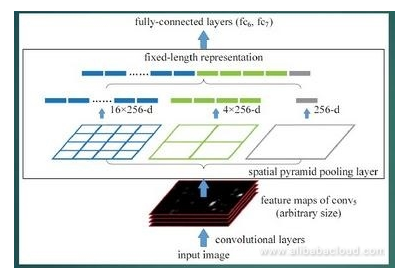2. Only extract convolutional features once for the original image
In R-CNN, each candidate box is resized to a standard size and then divided into CNN input, which reduces efficiency.

On the other hand, SPP Net only applies one convolution to obtain a full feature map, then finds the map patch of the zaifeature map of each candidate box, and treats this patch as convolutional feature input of the SPP layer and later layers. This saves a lot of computing time as it is a hundred times faster than R-CNN.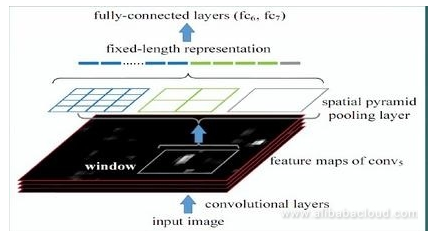## Fast R-CNN

SPP Net is a useful method. R-CNN's more advanced counterpart, Fast R-CNN, adopts the SPP Net method on the foundation of R-CNN, therefore improving performance.

So what are the differences between R-CNN and Fast R-CNN?

Let's first talk about the shortcomings of R-CNN. Even though it uses selective search and other preprocessing steps to extract the potential bounding box for input, R-CNN still has a serious speed bottleneck - the repeated computations will occur as the computer finds the features for each region.

Fast R-CNN was born to solve this specific issue.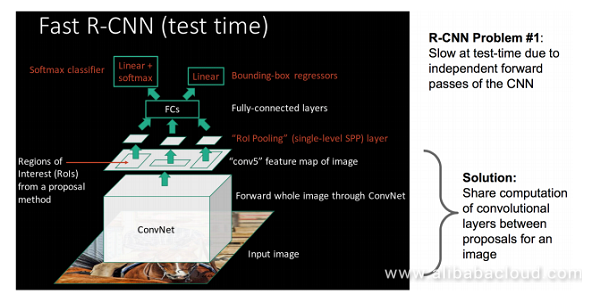In Fast R-CNN, there is a network layer called ROI Pooling similar to a single layer SPP Net. This network layer can map input of different sizes to a vector of a set length, so we can know that the conv, pooling, relu, and other operations don't need the input of a specific size. Therefore, after we have applied these operations to the original image, even though the varying size of the input images creates a feature map with a varying scale (meaning that we cannot directly connect them to a fully connected layer for classification), we can still add an impressive ROI Pooling layer. This layer then extracts a feature graph from each region according to a set dimension, then uses a normal softmax for category recognition.

Moreover, the processing path of the previous R-CNN was first to raise a proposal, then use the CNN to extract features, and next use the SVM classifier. Finally, perform bbox regression, and then, in the Fast R-CNN, the author deftly puts the bbox regression into the neural network and merges it with the region classification to form a multi-task model.

Experiments have proven that these two tasks are capable of sharing convolutional features and accelerate both. An essential contribution of Fast R-CNN is that it makes it possible to create a Region Proposal + CNN framework. It seems that multi-class inspections significantly increase processing speed while ensuring accuracy.

Let's summarize what we have discussed about R-CNN so far:

1. R-CNN has some glaring flaws (but once we get rid of these flaws, we are left with Fast R-CNN).
2. Major flaw: Because each candidate box needs to pass through the CNN independently, which is time-consuming.
3. Minor flaws: In the shared convolutional layer, not every candidate box enters the CNN as input. Instead, it enters as a complete picture and then retrieves the characteristics of each candidate box in the fifth convolutional layer.
4. Original method: Many candidate boxes (like two thousand) --> CNN--> get the features of each candidate box --> classification + regression.
5. Current method: a complete picture --> CNN --> get the features of each candidate box -> classification + regression.

It's easy to see why Fast R-CNN is so much faster than R-CNN; unlike R-CNN, which gives each candidate region to the deep network, the features are added to the entire map, and the candidate frame is mapped to conv5. SPP only needs to calculate the features once, the rest just need to operate on the conv5 layer.

Performance improvements are also quite obvious: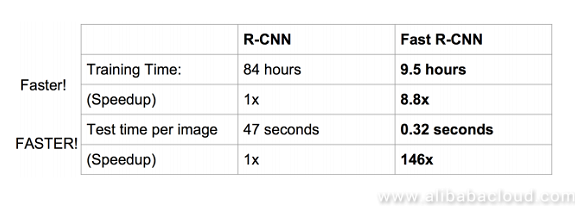## Faster R-CNN

There is no doubt that Fast R-CNN brings major improvements to traditional CNN. However, a major problem with Fast R-CNN is that it uses selective search to find all of the candidate boxes, which is quite time-consuming.

So is there a more efficient way to find these candidate boxes?

Solution: Add in a neural network that extracts edges. In other words, use the neural network to find the candidate boxes. The neural network that does this kind of task is called Region Proposal Network (RPN).

Let us look at a specific implementation of RPN:

1. Put the RPN behind the last convolution layer
2. The RPN trains directly to get the candidate area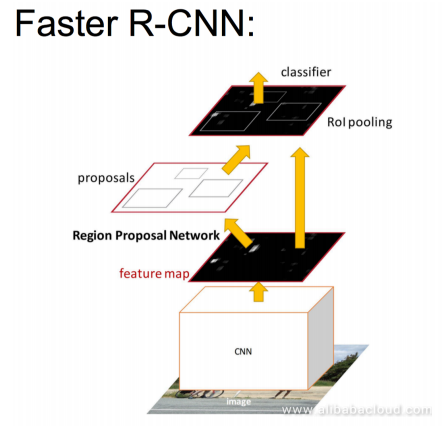RPN Summary:

1. Slide the window on the feature map.
2. Build a neural network for object classification + return the position of the box.
3. The position of the sliding window provides information about the general position of the object.
4. The return of the box offers a more precise position of the box.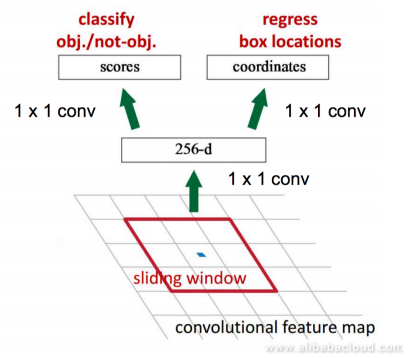One type of network with four loss functions:

1. RPN classification (anchor good.bad)
2. RPN regression(anchor -> proposal)
3. Fast R-CNN classification (over classes)
4. Fast R-CNN regression (proposal ->box)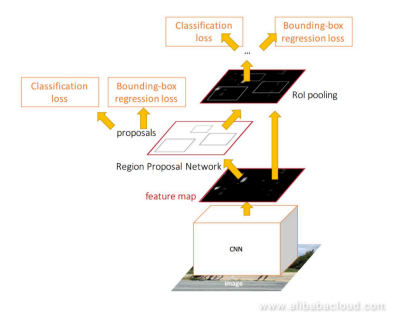Speed comparison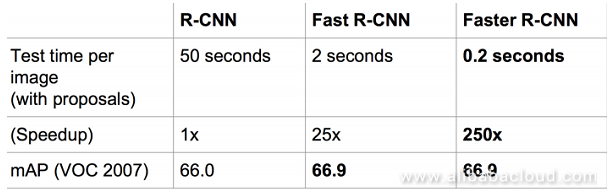The primary contribution of Faster R-CNN is that it designs a network RPN that extracts candidate areas instead of wasting time on selective search, which significantly accelerates the detection.

## Conclusion

In summary, having walked the path from R-CNN, SPP-NET, Fast R-CNN, and Faster R-CNN, the process of using deep learning target detection becomes more and more streamlined, accurate, and fast. We can say that target detection methods using R-CNN based on region proposal are currently the most prominent branch in the field of target detection technology.

Your machine learning project needs enormous amounts of training data to get to a production-ready confidence level. Get a checklist approach to assembling the combination of technology, workforce and project management skills you’ll need to prepare your own training data.

Topics:
artificial intelligence ,deep learning ,machine learning ,computer vision ,tutorial ,neural network

Comment (0)

Save
{{ articles.views | formatCount}} Views

Published at DZone with permission of

Opinions expressed by DZone contributors are their own.

# {{ parent.title || parent.header.title}}

{{ parent.tldr }}

{{ parent.urlSource.name }}